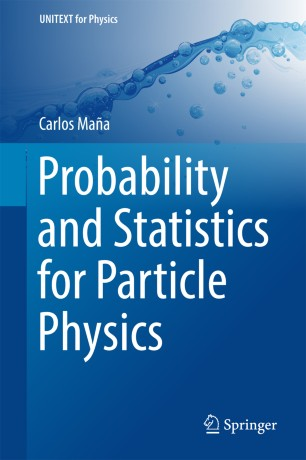# Probability and Statistics for Particle Physics

• Carlos MañaBook

Part of the UNITEXT for Physics book series (UNITEXTPH)

1. Front Matter
Pages i-x
2. Carlos Maña
Pages 1-85
3. Carlos Maña
Pages 87-167
4. Carlos Maña
Pages 169-220
5. Carlos Maña
Pages 221-244

### Introduction

This book comprehensively presents the basic concepts of probability and Bayesian inference with sufficient generality to make them applicable to current problems in scientific research.

The first chapter provides the fundamentals of probability theory that are essential for the analysis of random phenomena. The second chapter includes a full and pragmatic review of the Bayesian methods that constitute a natural and coherent framework with enough freedom to analyze all the information available from experimental data in a conceptually simple manner. The third chapter presents the basic Monte Carlo techniques used in scientific research, allowing a large variety of problems to be handled difficult to tackle by other procedures. The author also introduces a basic algorithm, which enables readers to simulate samples from simple distribution, and describes useful cases for researchers in particle physics.The final chapter is devoted to the basic ideas of Information Theory, which are important in the Bayesian methodology.

This highly readable book is appropriate for graduate-level courses, while at the same time being useful for scientific researches in general and for physicists in particular since most of the examples are from the field of Particle Physics.

### Keywords

Probability Measures Bayes Inference Integral Transforms Random Quantities Monte Carlo Methods Probability for particle physics Measure Theory Markov Chains Hypothesis Testing Decision theory Ordered Samples Limit Theorems Information Theory

#### Authors and affiliations

• Carlos Maña
• 1
1. 1.Departamento de Investigación BásicaCentro de Investigaciones Energéticas, Medioambientales y TecnológicasMadridSpain